### Mechanical Aptitude 5 - Gears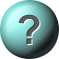1.    If Meshed gears have an unequal number of teeth then the gear with the fewest teeth?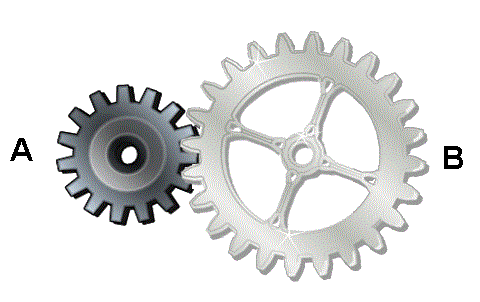will turn at equal speed.Will turn at different speed.Will turn faster.will turn slower.2.    In meshed gears to work out how fast one is turning with respect to the other you need to................?Count the teeth.See the direction of motion.See the size.Note the speed of each gear.3.    Gear A turns clockwise at a constant speed of 30 rpm, how does gear B turn, if the number of teeth in gear A are 8 and in gear B are 24?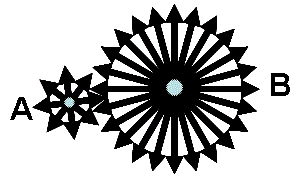Anti clockwise 30 rpmClockwise 10 rpmClockwise 5 rpmAnti clockwise 10 rpm4.    If Lower bar moves left at a constant speed, how does upper bar move?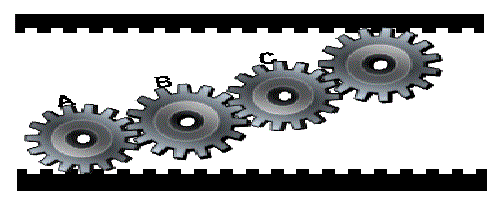Left, Slower than lower barRight, at the same speed as lower barLeft, Faster than lower barLeft, at the same speed as lower bar5.    Gear A turns clockwise at a constant speed of 10 rpm, how does gear B turn, if the number of teeth in each gear are equal?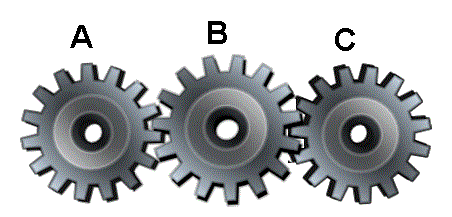Anti clockwise 10 rpmClockwise 20 rpmClockwise 10 rpmAnti clockwise 5 rpm

This is more feedback!
This is the feedback!